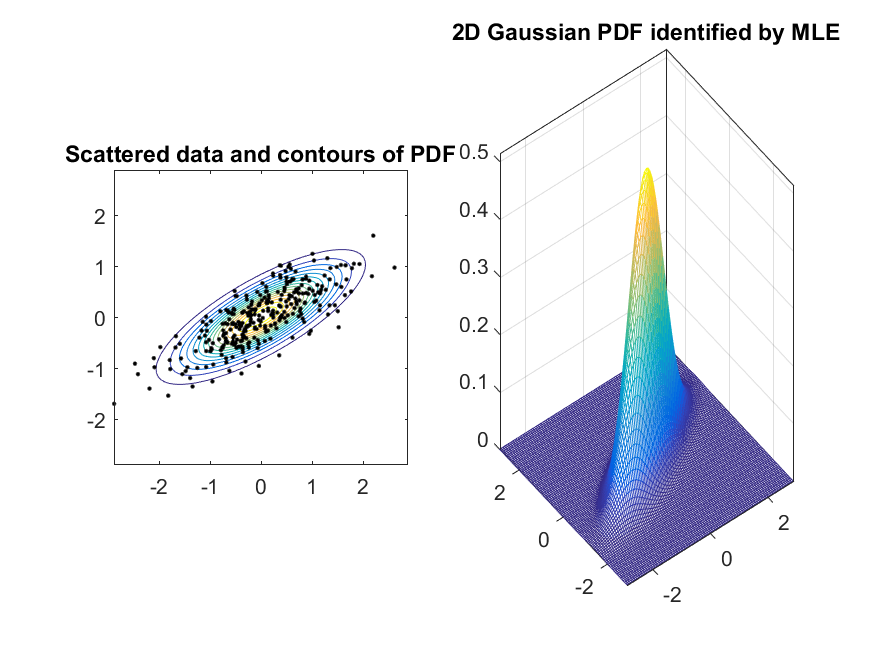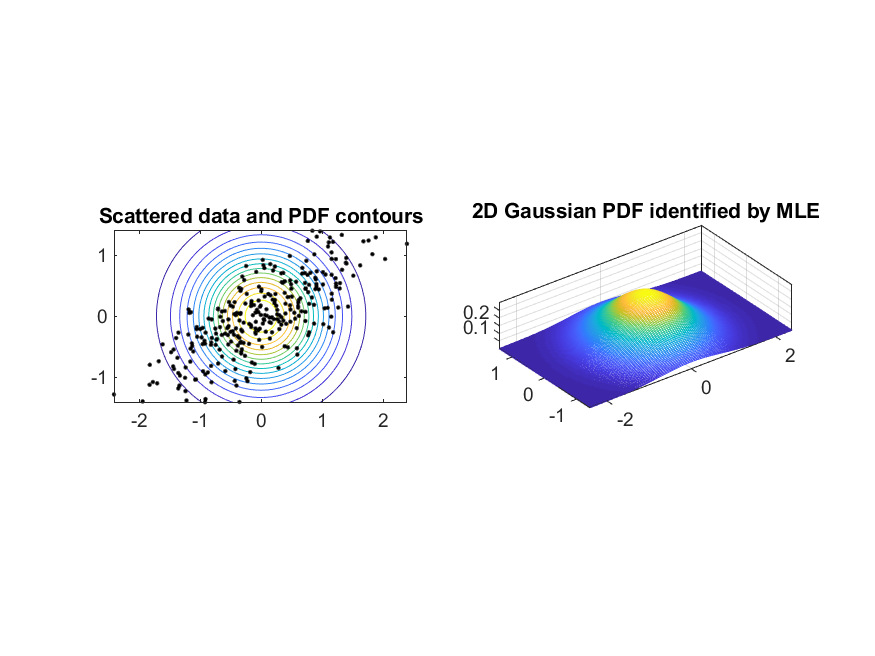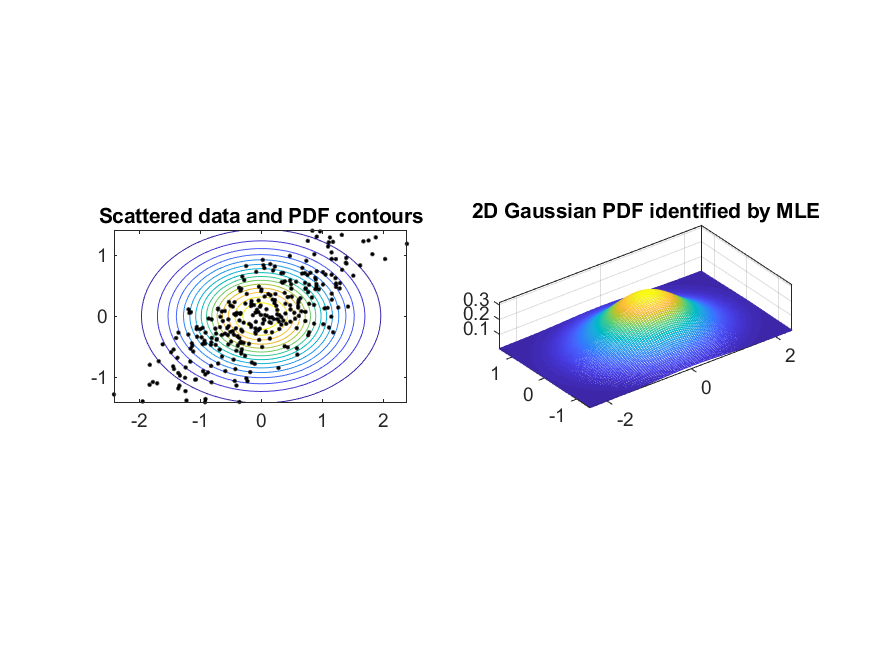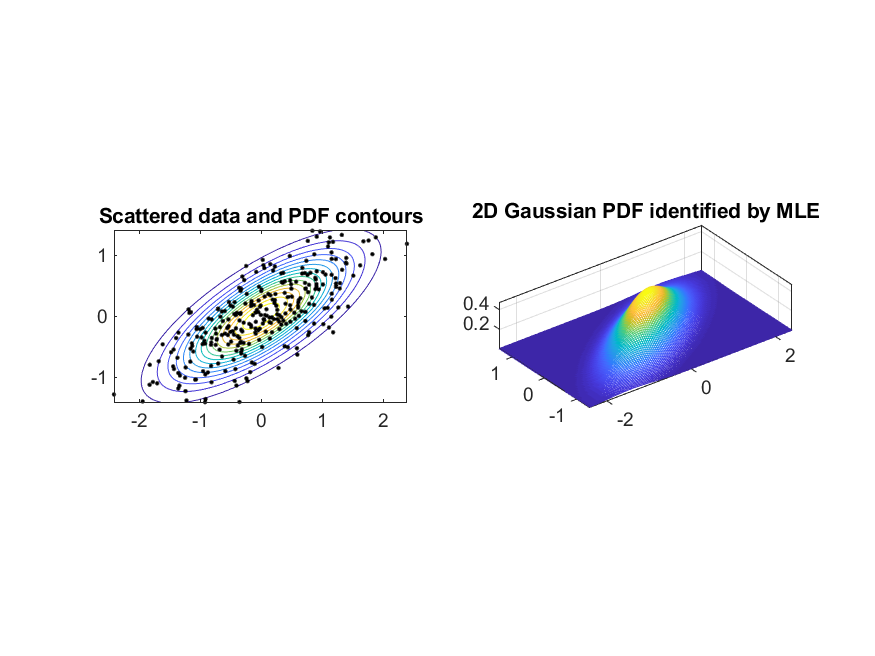4-4 PDF Modeling for ND Gaussian

Mathematical treatment can be found here.

Here is an example of MLE for 2D Gaussian PDF modeling:

Example 1: mle4gaussian02.mDS=dcData(7); gPrm=gaussianMle(DS.input); xMax=max(abs(DS.input(1,:))); yMax=max(abs(DS.input(1,:))); pointNum = 101; x = linspace(-xMax, xMax, pointNum); y = linspace(-yMax, yMax, pointNum); [xx, yy] = meshgrid(x, y); data = [xx(:), yy(:)]'; out = gaussian(data, gPrm); zz = reshape(out, pointNum, pointNum); subplot(1,2,1); contour(xx, yy, zz, 15); for i=1:size(DS.input,2) line(DS.input(1,i), DS.input(2,i), 'color', 'k', 'marker', '.'); end axis image; title('Scattered data and contours of PDF'); subplot(1,2,2); meshc(xx, yy, zz); axis([-inf inf -inf inf -inf inf]); set(gca, 'box', 'on'); title('2D Gaussian PDF identified by MLE');Actually we can use different types of covariance matrices for identifying the Gaussiaon PDF for a given dataset, including

1. Full covariance matrix
2. Diagonal covariance matrix
3. A single constant times an identity matrix
Example follows:

Example 2: mle4gaussian03.mds=dcData(7); showPlot=1; figure; gPrm=gaussianMle(ds.input, 'full', showPlot); figure; gPrm=gaussianMle(ds.input, 'diagonal', showPlot); figure; gPrm=gaussianMle(ds.input, 'single', showPlot);Data Clustering and Pattern Recognition (資料分群與樣式辨認)